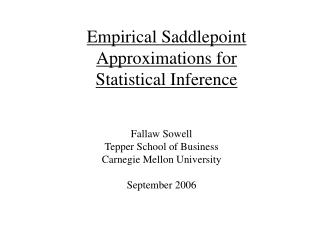Download PresentationEmpirical Saddlepoint Approximations for Statistical Inference# Empirical Saddlepoint Approximations for Statistical Inference - PowerPoint PPT Presentation

Download Presentation##### Empirical Saddlepoint Approximations for Statistical Inference

Download Policy: Content on the Website is provided to you AS IS for your information and personal use and may not be sold / licensed / shared on other websites without getting consent from its author. While downloading, if for some reason you are not able to download a presentation, the publisher may have deleted the file from their server.

- - - - - - - - - - - - - - - - - - - - - - - - - - - E N D - - - - - - - - - - - - - - - - - - - - - - - - - - -
##### Presentation Transcript

1. Empirical Saddlepoint Approximations forStatistical Inference Fallaw Sowell Tepper School of Business Carnegie Mellon University September 2006

2. Basic Issues • More accurate statistical inference for better decisions. • Most important in nonlinear models and/or situations • where there is only a small amount of data. • Computational intensive procedure to give an improved • approximations to the sampling distributions. • Converges to the familiar asymptotic normal distribution • in “large” samples. • Allows multiple modes, asymmetric distribution, • non-normal tail behavior, relative convergence.

3. Basic Intuition • The traditional asymptotic Normal approximations • use local information about the objective function • at one point to create an approximation over the • entire parameter space. • The empirical saddlepoint approximation uses • information about the global shape of the objective • function to create an approximation over the entire • parameter space. • Instead of one linear approximation to the objective • function, combine a continuum of linear approximations.

4. Traditional Approach (MLE) FOC’s Perform a linear approximation. Apply a central limit theorem

5. Traditional Approach (GMM) FOC’s Perform a linear approximation. Apply a central limit theorem

6. Traditional Approach Intuition 1. A multivariate normal distribution with the mean at the location of the extreme value and covariance given by the convexity at the extreme value. 2. Linear approximation to the FOC’s. 3. Equivalent to a quadratic approximation to the objective function, an elliptic paraboloid.

7. Hall and Horowitz (Econometrica,96) Look at some sampling distributions, various values of N.

8. Saddlepoint Approximation Intuition -Measures of convexity at -Local minima associated with

9. Steps to use this in practice 1. GMM moments to create estimation equations. 2. Determine the local minima. 3. Over a grid of values calculate the convexity. (Solve m equations in m unknowns.) 4. Numerically integrate to obtain marginal densities.

10. Rate of convergence • Convergence is relative. relative absolute • Relative implies • Better tail approximation compared to • asymptotic and bootstrap approximations.